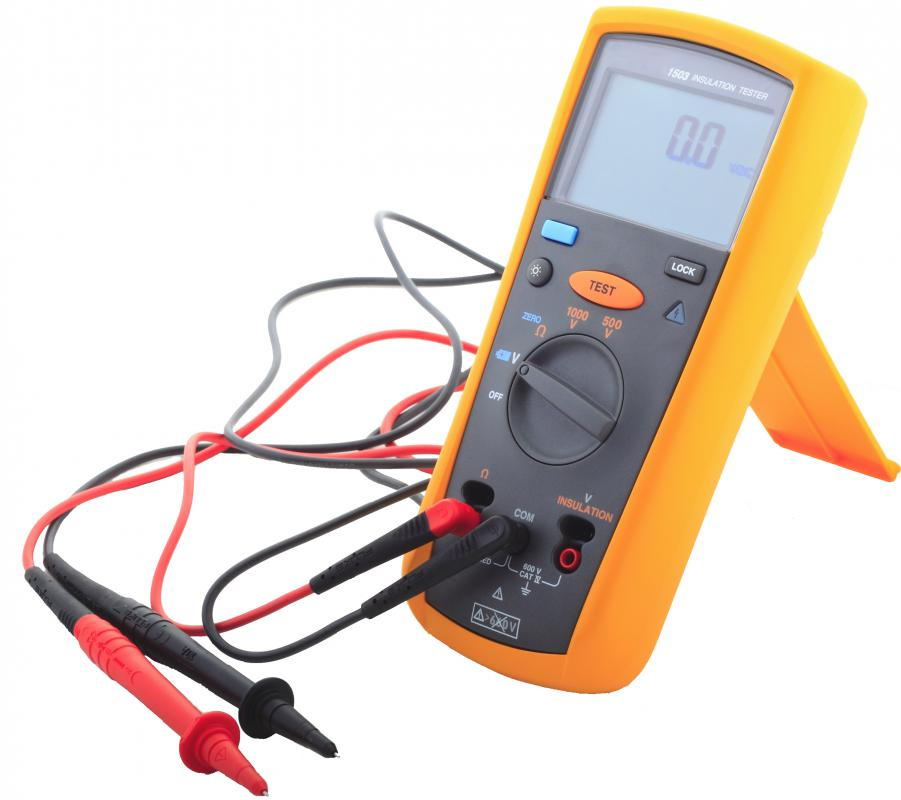# What Are the Different Types of Multimeters?

Jerry Morrison

There are two primary types of multimeter: analog and digital. Each is designed to measure the same basic electrical values, but differ in their method of measurement and display. The analog ones were developed first, and rely on a moving pointer over a graduated scale to indicate readings. Digital meters typically use a Liquid Crystal Display (LCD) or Light Emitting Diodes (LED) to display readings numerically. Multimeters are widely used and implementations within each device type might vary considerably according to the specific measurements to be made, the accuracy required, and the work environment.A digital multimeter is a tool that can measure amps, volts, and ohms.

Analog multimeters are built around an ammeter, a device used to measure electrical current in amperes, the basic unit of electrical measurement. Current from a circuit being measured passes through a metal coil in the presence of a magnetic field. Attached to the coil is a pointer whose angle of rotation is proportional to the strength of the current. Measured values are indicated as the pointer passes over a range of graduated scales on the face of the meter. These devices are electromechanical, converting electrical to mechanical energy.

Digital multimeters use digital circuits, which measure current as discrete increments rather than as a continuous range of values. Since digital circuits are made from analog components, continuous signals must be converted into digital form. In digital meters, this is accomplished by converting the input signal into a voltage and strengthening the signal for further processing. Though inherently more accurate than analog meters, the time factor involved in processing the signal often makes digital devices unsuitable for measuring values that are in constant change.

Each type of multimeter typically measures current, voltage and resistance. The digital kind often provides further capabilities such as measuring capacitance, a measure of stored charge and potential, and diode testing. Since they produce digital data, they can be directly interfaced with a computer or their data may be later transferred for storage and further analysis.

Both analog and digital multimeters are designed to measure direct current (DC) only and must be modified to measure alternating current (AC). Typically, this requires incorporating a rectifier circuit, which converts AC to DC, and averaging periodic measurements of the current. The average is used to calculate the root mean square (RMS) which is reported as the AC value. A digital subtype, called true RMS multimeters, use a more accurate calculation derived from the heat dissipated by the current against a constant resistive load.

## You might also Like